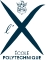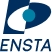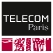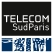Course Finder

PhD

Applied Mathematics

Field(s) : Applied mathematics, statistics Mathematics and applications
Specialtie(s) : Applied algebra Applied analysis Numerical analysis Scientific computing Applied geometry Mathematical engineering Financial mathematics Modeling Optimization Applied probability Applied statistics
Duration : 3 years
Language(s) : French English
Bachelor of science

Mathematics and Computer Science

Field(s) : Economics Applied mathematics, statistics Mathematics and applications
Specialtie(s) : Applied mathematics, statistics Mathematics and applications Computer Science
Duration : 3 years
Language(s) : English
Bachelor of science

Mathematics and Economics

Field(s) : Applied mathematics, statistics Mathematics and applications Mechanics Physics
Specialtie(s) : Applied mathematics, statistics Mathematics and applications Economics
Duration : 3 years
Language(s) : English
Bachelor of science

Mathematics and Physics

Field(s) : Computer science Applied mathematics, statistics Mathematics and applications
Specialtie(s) : Applied mathematics, statistics Mathematics and applications Mechanics Physics
Duration : 3 years
Language(s) : English
PhD

Mathematics at Interfaces

Field(s) : Applied mathematics, statistics Mathematics and applications
Specialtie(s) : Mathematics-economics Mathematics-IT Mathematics-mechanical engineering Mathematics-physics Mathematics-engineering science Mathematics-life sciences
Duration : 3 years
Language(s) : French English
PhD

Physics

Field(s) : Physics
Specialtie(s) : Optics Laser Plasma Astrophysics Condensed matter physics Particle physics Quantum physics
Duration : 3 years
Language(s) : French English
PhD

Pure Mathematics

Field(s) : Applied mathematics, statistics Mathematics and applications
Specialtie(s) : Algebra Analysis Geometry Logic Probability Statistics
Duration : 3 years
Language(s) : French English